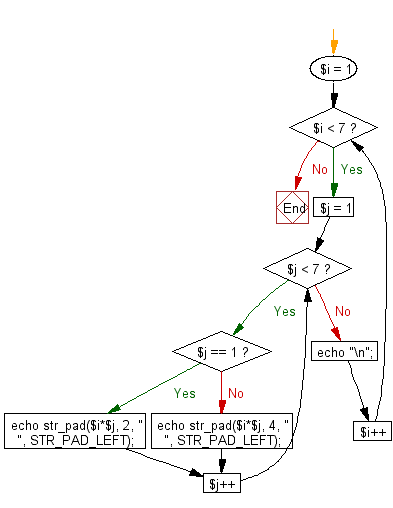﻿ PHP Exercise: Print out the multiplication table upto 6*6 - w3resource

# PHP Exercises: Print out the multiplication table upto 6*6

## PHP: Exercise-41 with Solution

Write a PHP program to print out the multiplication table upto 6*6.

Sample Solution: -

PHP Code:

``````<?php
for (\$i = 1; \$i < 7; \$i++) {
for (\$j = 1; \$j < 7; \$j++) {
if (\$j == 1) {
} else {
}
}
echo "\n";
}
?>
```
```

Sample Output:

```1   2   3   4   5   6
2   4   6   8  10  12
3   6   9  12  15  18
4   8  12  16  20  24
5  10  15  20  25  30
6  12  18  24  30  36
```

Flowchart:PHP Code Editor:

Have another way to solve this solution? Contribute your code (and comments) through Disqus.

What is the difficulty level of this exercise?

Test your Programming skills with w3resource's quiz.

﻿

## PHP: Tips of the Day

Mutates the original array to filter out the values specified

Example:

```<?php
function tips_pull(&\$items, ...\$params)
{
\$items = array_values(array_diff(\$items, \$params));
return \$items;
}

\$items = ['x', 'y', 'z', 'x', 'y', 'z'];
print_r(tips_pull(\$items, 'y', 'z'));
?>
```

Output:

```Array
(
 => x
 => x
)
```